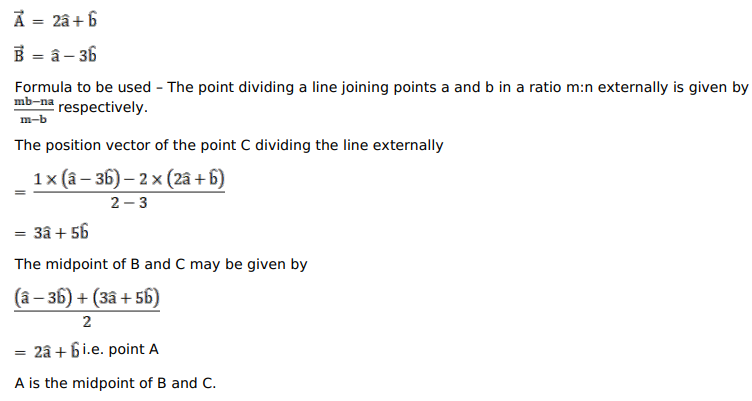# The position vectors of two points

Question:

The position vectors of two points $A$ and $B$ are $(2 \vec{a}+\vec{b})$ and $(\vec{a}-3 \vec{b})$ respectively. Find the position vector of a point $C$ which divides $A B$ externally in the ratio $1: 2$. Also, show that $A$ is the mid-point of the line segment CB.

Solution: